NVIDIA 2D Image And Signal Performance Primitives (NPP)  Version 11.3.0.*

Primitives for computing the norms of an image, the norms of difference, and the relative errors of two images. More...

## Modules

Norm_Inf
Primitives for computing the infinity norm of an image.

Norm_L1
Primitives for computing the L1 norm of an image.

Norm_L2
Primitives for computing the L2 norm of an image.

NormDiff_Inf
Primitives for computing the infinity norm of difference of pixels between two images.

NormDiff_L1
Primitives for computing the L1 norm of difference of pixels between two images.

NormDiff_L2
Primitives for computing the L2 norm of difference of pixels between two images.

NormRel_Inf
Primitives for computing the relative error of infinity norm between two images.

NormRel_L1
Primitives for computing the relative error of L1 norm between two images.

NormRel_L2
Primitives for computing the relative error of L2 norm between two images.

## Detailed Description

Primitives for computing the norms of an image, the norms of difference, and the relative errors of two images.

Given an image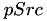with width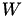and height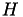,

1. The infinity norm (Norm_Inf) is defined as the largest absolute pixel value of the image.
2. The L1 norm (Norm_L1) is defined as the sum of the absolute pixel value of the image, i.e.,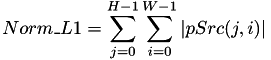.
3. The L2 norm (Norm_L2) is defined as the square root of the sum of the squared absolute pixel value of the image, i.e.,.

Given two images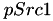and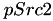both with widthand height,

1. The infinity norm of differece (NormDiff_Inf) is defined as the largest absolute difference between pixels of two images.
2. The L1 norm of differece (NormDiff_L1) is defined as the sum of the absolute difference between pixels of two images, i.e.,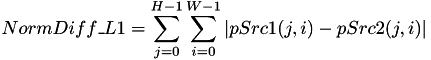.
3. The L2 norm of differece (NormDiff_L2) is defined as the squared root of the sum of the squared absolute difference between pixels of two images, i.e.,.

Given two imagesandboth with widthand height,

1. The relative error for the infinity norm of differece (NormRel_Inf) is defined as NormDiff_Inf divided by the infinity norm of the second image, i.e.,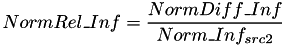2. The relative error for the L1 norm of differece (NormRel_L1) is defined as NormDiff_L1 divided by the L1 norm of the second image, i.e.,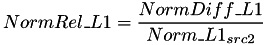3. The relative error for the L2 norm of differece (NormRel_L2) is defined as NormDiff_L2 divided by the L2 norm of the second image, i.e.,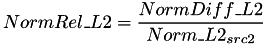The norm functions require the addition device scratch buffer for the computations.

### Common parameters for nppiNorm functions include:

Parameters
 pSrc1 Source-Image Pointer. nSrc1Step Source-Image Line Step. pSrc2 Source-Image Pointer. nSrc2Step Source-Image Line Step. oSizeROI Region-of-Interest (ROI). pMask Mask-Image Pointer. nMaskStep Mask-Image Line Step. nCOI Channel_of_Interest Number. pNorm Pointer to the norm value. pNormDiff Pointer to the computed norm of differences. pNormRel Pointer to the computed relative error for the infinity norm of two images. pDeviceBuffer Pointer to the required device memory allocation, Scratch Buffer and Host Pointer. Use nppiNormInfGetBufferHostSize_XX_XXX to compute the required size (in bytes). nppStreamCtx Application Managed Stream Context.
Returns
Image Data Related Error Codes, ROI Related Error Codes, or NPP_COI_ERROR if an invalid channel of interest is specified, or NPP_NOT_EVEN_STEP_ERROR if an invalid floating-point image is specified.